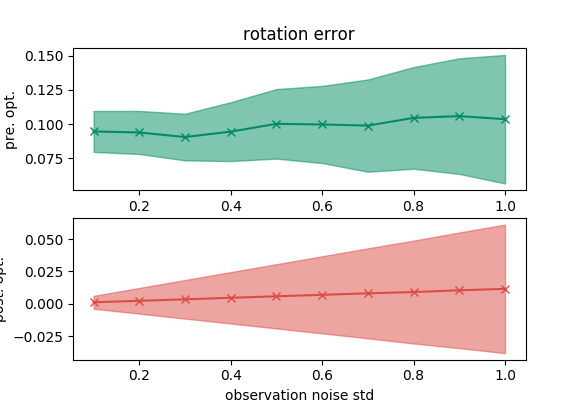07 May

#### Tests on the modules of optimization problems

The optimization based SLAM formulate the optimization problem with two kinds of constraints. First, the pre-integrated IMU error establishes the constraints between two spatial states, or two poses. The other one is the reprojection error that links a spatial state and a landmark. In the optimization tool, we have to specify the Jacobian of the error, which tells the optimization tool which direction to make the entire cost smaller. While both the pre-integration model and the reprojection model are nonlinear, these two blocks are prone to error.

We develop the following two simple test schemes, modified from the okvis project, in order to make sure the these modules in our system is correct.

All the implementation can be found in my github repo.

## Pre-integrated IMU error

In this test simulation, we generate the IMU data with 1kHz over a 0.1 sec duration, and we vary the noise density of the IMU measurements.

We first take the correct initial state and set it constant in the optimization problem. We then add some noise to the final state to see whether the optimization tool can successfully recover the correct final state based on the pre-integrated IMU data.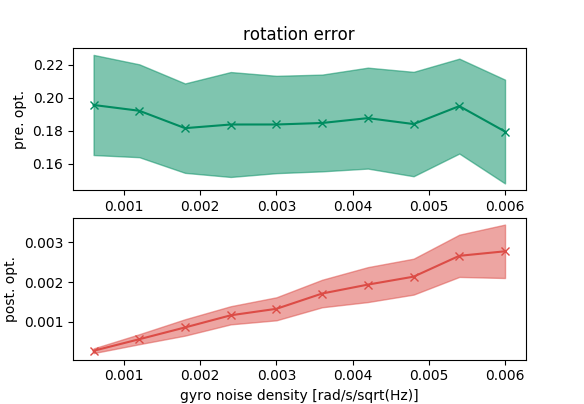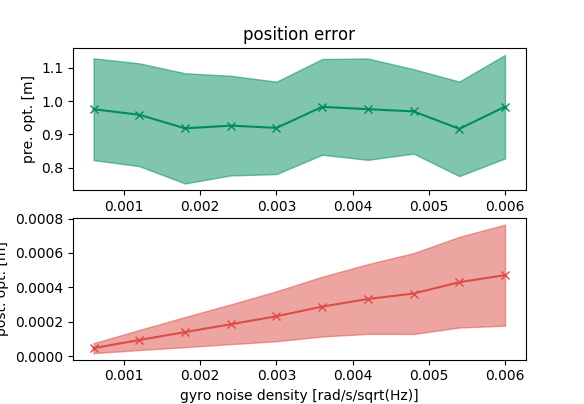From the previous plots, we can see that the optimization tool can recover the final state. Furthermore, the errors of the recovered state grow with the IMU errors.

Now, we switch the role of the initial state and the final state. We use the correct final state in the optimization problem and set it constant to see if the optimization problem can find the correct initial state.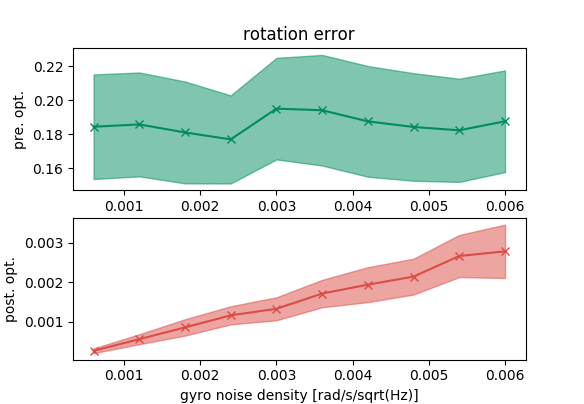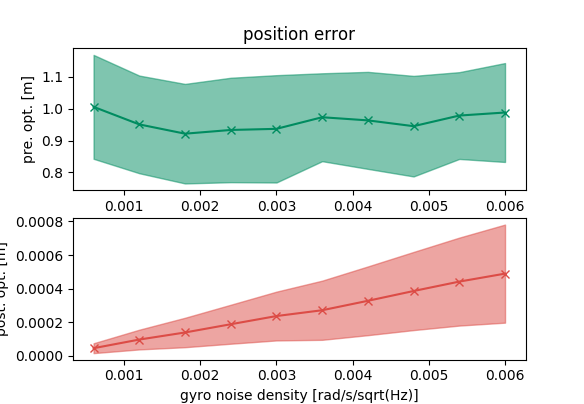We observe the similar trend in this scenario that the optimization tool is correct.

## Reprojection error

To test reprojection error, we randomly generate a pose, and then generate 100 random 3D points that the pose can observe. We then project those 100 points on the camera with that pose. In the optimization problem, we have 100 reprojection constraints. We set the correct landmarks in the optimization problem, and add random disturbance to the pose. The optimization tool is supposed to recover the pose.

We vary the observation noise and get the following plots, which show that the optimization tool does correctly to recover the pose.